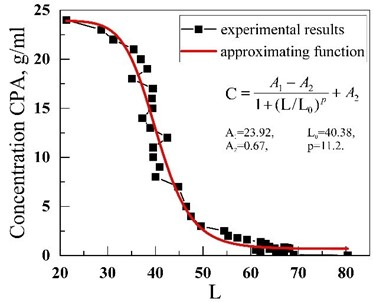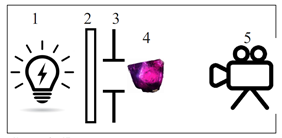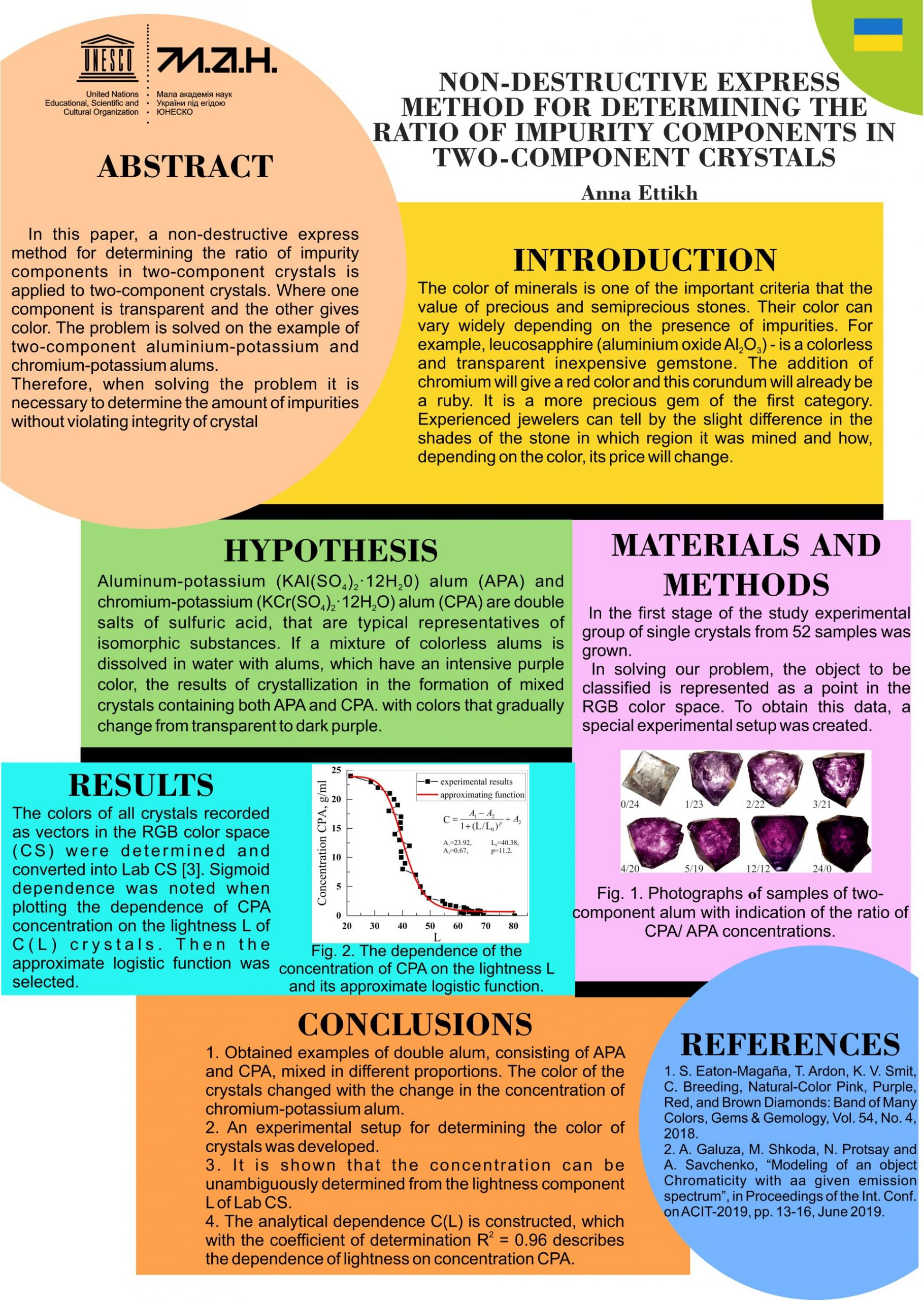# Non-destructive express method for determining the ratio of impurity components in two-component crystals

•••••Author: ANNA ETTIKH

Menthor: ALLA SAVCHENKO

School: UNESCO Centre “Junior Academy of Science of Ukraine” (JASU)

In this paper, a non-destructive express method for determining the ratio of impurity components in two-component crystals is proposed. The method is applied to two-component crystals, where one component is transparent and the other gives color. The problem is solved on the example of two-component aluminum-potassium alum (APA) and chromium-potassium alum (CPA).

At the first stage of the study, the method of concentration by convection of aqueous supersaturated solutions was used for growing crystals. They contain mixtures of APA and HPA, taken in different proportions (24 g of alum per 100 g of water at a ratio of components x / (24-x, where x is the mass of HPA) grown experimental set of single crystals from 52 samples.

To determine the color of the crystals from digital photography, a special experimental setup was created that provided uniform lighting and the same shooting conditions for all objects. Using the obtained photographs, the color of each crystal was determined as a point with coordinates (R, G, B) in the RGB color space (CS) and a 3D graph was constructed, the nature of which turned out to be very chaotic. Analytical dependence could not be obtained for any color component. It was decided to move to another CS CIELab. The coordinates of each crystal were converted from RGB to Lab CS and a 3D graph was constructed. A tendency to decrease the lightness coordinate L was immediately noticed. When plotting the dependence of all three color components L, a, b on the concentration, a pronounced sigmoid nature of the luminosity dependence of crystals on the HPA concentration L (C) was observed. Therefore, an approximate logistics function was chosen. Coefficient of determination R2=0.96, therefore, this function describes the experimental results quite well.

So, in the study:

1. Obtained samples of double alum, consisting of aluminum-potassium (KAl(SO4)2·12H2O) and chromium-potassium (KCr(SO4)2·12H2O) alum, mixed in different proportions. The color of the crystals changed with the change in the concentration of chromium potassium alum.
2. An experemental setup for determining the color of crystals was developed and the dependences of the color of crystals on the concentration of KCr(SO4)2·12H2O in different CPs were investigated.
3. It is shown that the concentration can be unambiguously determined from the lightness component L Lab CS.
4. The analytical dependence C (L) is constructed, which with the coefficient of determination is equal to R2 = 0.96 describes the dependence of light on concentration.
5. This approach can be used to determine the concentrations of other two-component crystals. To do this, we need to obtain a new analytical dependence C (L).